# Parametric representation of univalent functions

(diff) ← Older revision | Latest revision (diff) | Newer revision → (diff)

A representation of univalent functions (cf. Univalent function) that effect a conformal mapping of planar domains onto domains of a canonical form (for example, a disc with concentric slits); it usually arises in the following manner. One chooses a one-parameter family of domains,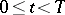, included in one another,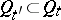,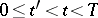. For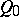one assumes known a conformal mapping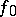onto some canonical domain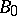. From a known mapping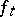ofonto a domain of canonical form one constructs such a mapping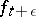for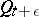, whereis small. Under a continuous change of the parameterthere arise in this way differential equations. The best known of these are the Löwner equation and the Löwner–Kufarev equation. In the discrete case — for lattice domains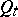and a natural number— the transition fromto,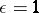, is effected by recurrence formulas. The source of these formulas and equations is usually the Schwarz formula (see ) and its generalizations (see ). An equally important source of parametric representations are the Hadamard variations (see , ) for the Green functions,, of the family of domains mentioned above. For elliptic differential equations Hadamard's method is also called the method of invariant imbedding (see ). Below, the connection between parametric representations, Hadamard variations and invariant imbedding are exhibited in the simplest (discrete) case.

Suppose thatis a collection of complex integers (a lattice domain) and that the Green functionis an extremal of the Dirichlet–Douglas functional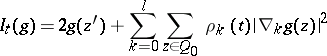in the classof all real-valued functionson. Here,(1)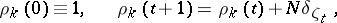is a natural number,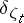is the Kronecker symbol, and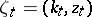,, is a certain collection of pairs of numbers;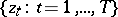is the boundary of, and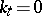or 1. To find an extremum of the functional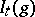is a problem of quadratic programming. A comparison of its solutions forand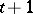gives the basic formula of invariant imbedding (Hadamard variation):(2)

where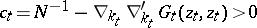and the symbol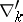denotes the difference operators (1) in the second argument of the Green function. Knowingone can obtain step-by-step (recurrently) from (2) all the functions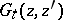,. By constructing the Green function, one obtains from the lattice analytic function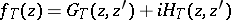according to the equation of Cauchy–Riemann typea univalent lattice quasi-conformal mapping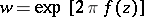ofinto the unit disc. Closest to the origin of coordinates is the image of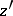. In the limit, as, the mapping is lattice conformal and the image of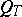is a disc with concentric slits. The result is a continuous analogue of (2) (see ). When all the domains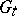are simply connected and the canonical domain is the unit disc, one succeeds by using a fractional-linear automorphism ofto represent the Green function in the explicit formin terms of the function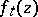mappingontowith the normalization,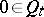for all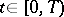.

In terms of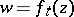the Hadamard variation reduces to an ordinary (Löwner) differential equation. In comparison with the Hadamard variation this equation is considerably simpler; however, information on the boundary ofis only implicit in it — in terms of the control parameter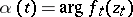, because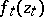is not known beforehand. Nevertheless, the Löwner equation is a basic instrument in the parametric representation.

More general one-parameter families of domains,, not necessarily imbedded in one another, have also been treated (see ). The equations arising in such parametric representations are called Löwner–Kufarev equations. There is also a modification of the Löwner and Löwner–Kufarev equations to the case when the domainshave a different kind of symmetry or other geometric peculiarities (see ).

How to Cite This Entry:
Parametric representation of univalent functions. Encyclopedia of Mathematics. URL: http://encyclopediaofmath.org/index.php?title=Parametric_representation_of_univalent_functions&oldid=18588
This article was adapted from an original article by V.I. Popov (originator), which appeared in Encyclopedia of Mathematics - ISBN 1402006098. See original article